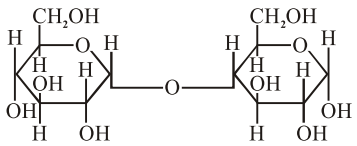# Which of the following is correct`
Question:

Which of the following is correct structure of $\alpha$-anomer of maltose ?

1.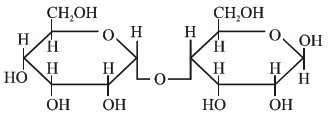2.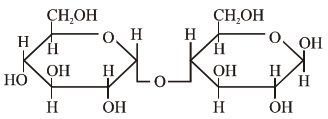3.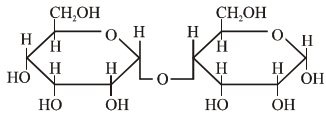4.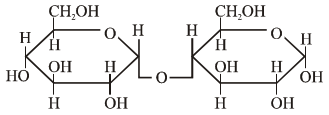Correct Option: , 4

Solution:

$\alpha-A N O M E R$ OF MALTOSE

maltose is disaccharides of $\alpha-\mathrm{D}-$ glucopyranose by $\mathrm{C}_{1}-\mathrm{C}_{4}$ glycosidic linkage